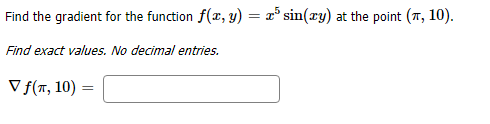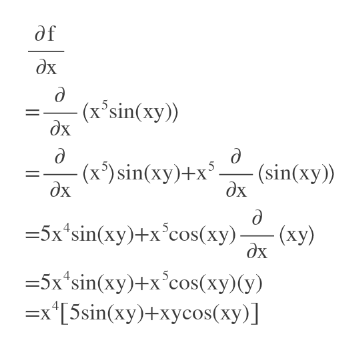# Find the gradient for the function f(x, y) = x5 sin(xy) at the point (, 10)Find exact values. No decimal entries.Vf(T, 10)

Question
1 viewshelp_outlineImage TranscriptioncloseFind the gradient for the function f(x, y) = x5 sin(xy) at the point (, 10) Find exact values. No decimal entries. Vf(T, 10) fullscreen
check_circle

Step 1

To find the gradient of a function (which is a vector), differentiate the function with respect to each variable.

Step 2

Find partial derivative of f w.r.t. xhelp_outlineImage Transcriptioncloseдf дх д (х'sin(xy)) дх д е (sin(xy) (х) sin(xy)+x5. дх дх д %35x'sin(xy)+x'cos(ху). (ху) дх 35x'sin(xy)+x'cos(ху) (у) —х"[5sin(xy)+xycos(ху)] fullscreen
Step 3

Find partial derivative of f ...

### Want to see the full answer?

See Solution

#### Want to see this answer and more?

Solutions are written by subject experts who are available 24/7. Questions are typically answered within 1 hour.*

See Solution
*Response times may vary by subject and question.
Tagged in

### Other